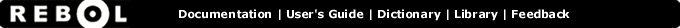# In - Function Summary

## Summary:

Returns the word in the object's context.

in object word

## Arguments:

object - The object argument. (must be: object port)

word - The word argument. (must be: any-word)

## Description:

Return the word from within another context. This function is normally used with SET and GET.

 ``` set-console: func ['word value] [ set in system/console word value ] set-console prompt "==>" set-console result "-->" ```

This is a useful function for accessing the words and values of an object. The IN function will obtain a word from an object's context. For example, if you create an object:

 ``` example: make object! [ name: "fred" age: 24 ] ```

You can access the object's name and age fields with:

 ``` print example/name print example/age fred 24```

But you can also access them with:

 ``` print get in example 'name print get in example 'age fred 24```

The IN function returns the NAME and AGE words as they are within the example object. If you type:

 ``` print in example 'name name```

The result will be the word NAME, but with a value as it exists in the example object. The GET function then fetches their values. This is the best way to obtain a value from an object, regardless of its datatype (such as in the case of a function).

A SET can also be used:

 ``` print set in example 'name "Bob" Bob```

Using IN, here is a way to print the values of all the fields of an object:

 ``` foreach word next first example [ probe get in example word ] "Bob" 24```

The FIRST of the object returns the list of words within the object. The NEXT skips the first word, which is SELF, the object itself.

Here is another example that sets all the values of an object to NONE:

 ``` foreach word next first example [ set in example word none ] ```

The IN function can also be used to quickly check for the existence of a word within an object:

 ``` if in example 'name [print example/name] none```

 ``` if in example 'address [print example/address] ```

This is useful for objects that have optional variables.

## Related:

get - Gets the value of a word.
set - Sets a word or block of words to specified value(s).